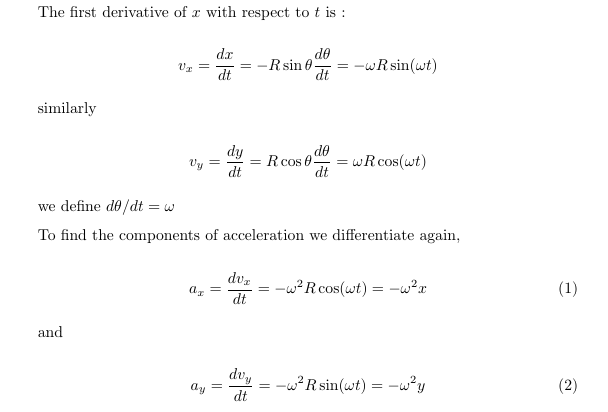# A particle is moving around in a circle and its position is given in polar coordinates as x = Rcosθ, and y = Rsinθ, where R is the radius of the circle, and θ is in radians. From these equations derive the equation for centripetal acceleration.

Question
14 views

A particle is moving around in a circle and its position is given in polar coordinates as x = Rcosθ, and y = Rsinθ, where R is the radius of the circle, and θ is in radians. From these equations derive the equation for centripetal acceleration.

check_circle

Step 1...

### Want to see the full answer?

See Solution

#### Want to see this answer and more?

Solutions are written by subject experts who are available 24/7. Questions are typically answered within 1 hour.*

See Solution
*Response times may vary by subject and question.
Tagged in